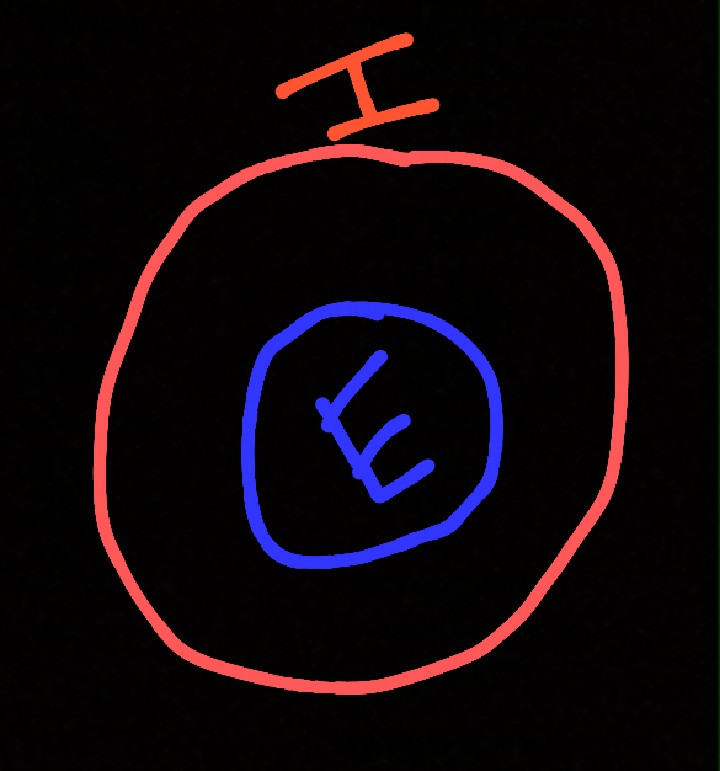Forums

# Sets

I is set of isosceles triangles and E is the set of equilateral triangles,then what will be the answer 1) I is the subset of E or 2) E is the subset of I On my POV, 1) shall be the answer, because not all isosceles triangle are equilateral whereas all equilateral triangles are isosceles; but the given answer is 2) Kindly clarifyYamuna S

CA Foundation

4K+

23-Sep-22

97

Aswin Chandar

Hey there, you have the correct explanation but just that you have mistaken the interpretation. Isosceles triangles are the bigger circle/main category, under that equilateral is the sub-category. So clearly E is the subset of I and not otherwise.

Just for more clarity.Aswin Chandar

CA Inter

18K+

23-Sep-22

Subset means all the elements in it are present in the superset. Then it is called as a subset. It we take equilateral triangle, it possesses the characteristics of isosceles triangle. But isosceles triangle don't(it means isosceles triangle don't have characteristics of an equilateral triangle). Hence, equilateral triangle is subset of isosceles triangle.Rupa Devi Akkala

CA Foundation

23K+

23-Sep-22

Hey there, you have the correct explanation but just that you have mistaken the interpretation. Isosceles triangles are the bigger circle/main category, under that equilateral is the sub-category. So clearly E is the subset of I and not otherwise.Aswin Chandar

CA Inter

18K+

23-Sep-22

Does sets form part of ca fnd maths syllabus?Dhakshana Dhakshana

CA Inter

16K+

23-Sep-22

Dhakshana Dhakshana

Does sets form part of ca fnd maths syllabus?

Yes they do.Aswin Chandar

CA Inter

18K+

23-Sep-22

Thanks all.. got it!Yamuna S

CA Foundation

4K+

23-Sep-22

Yamuna S

Thanks all.. got it!

Glad we could help.Aswin Chandar

CA Inter

18K+

23-Sep-22

Sales